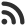Feed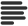Articles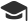Tutorials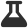Lab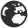CompaniesLeaderboard
 DG Student at BIT Mesra Aug. 18, 2020, 9:52 a.m. ⋅ 21173 views

# CapGemini Pseudo Code Questions with Answer and Explanation (Set 1)

This is set 1 of CapGemini Pseudo Code Questions.

Set 2 Can be found from here: https://www.hackersfriend.com/articles/capgemini-pseudo-code-questions-with-answer-and-explanation-set-2

Set 3 can be found from here: https://www.hackersfriend.com/articles/capgemini-pseudo-code-questions-with-answer-and-explanation-set-3

1. What will be the output of following code :

``````#include<stdio.h>
int main()
{
int num = 8;
printf (“%d %d”, num << 1, num >> 1);
return 0;
}``````

A. 8 0
B. 0 0
C. 16 4
D. Error : Can’t Perform operation

Ans. C

Explanation : ‘<<‘ This is the left shift operator. It takes two numbers, left shifts the bits of the first operand, the second operand decides the number of places to shift. ‘>>’ This is the right shift operator.

8 in binary form : 00001000

Performing Left Shift (<<) : 00010000 => 16

Performing Right Shift (>>) : 00000100 => 4

2. What is the output of given code :

``````#include<stdio.h>
int main()
{
long double a;
long double b;
int arr[sizeof(!a+b)];
printf(“%d”,sizeof(arr));
}``````

A. Run time Error
B. 32
C. 64 with warning
D. No output

Ans. C

Explanation : Size of long double in GCC Linux based compilers : 16

Size of Integer : 4

So, Size of !(a+b) : 16

Integer array of size 16 : 16 * 4 => 64

3. Which of the following statements is true regarding, Auto Storage Class ?

A. It is used to give a reference of a global variable that is visible to all program files.
B. It instructs the compiler to keep a local variable in existence during the lifetime of a program.
C. It is the default storage class for all local variables.
D. It is used to define local variables that should be stored in a register.

Ans. C

4. What will be the output of the following code :

``````integer a = 984, b=10
float c
c = a / b
print c``````

A. 984
B. 98.4
C. 98
D. Error

Ans. C

Explanation : Answer will be like 98.0000, here C is the most suited option.

5. What is the output of given code (Input : 1.45):

``````#include<stdio.h>
int main()
{
int ch;
print(“Enter a value between 1 & 2”);
scanf(“%d”, &ch);
switch(ch, ch+1)
{
case 1 :
printf(“1\n”);
break;
case 2 :
printf(“2\n”);
break;
default :
printf(“3\n”);
}``````

A. 1
3
B. Error : Undefined condition in switch
C. 2
D. No output

Ans. C

Explanation : For ch = 1.45,

switch() will be executed for ch=2, and hence 2 will be printed as o/p.

6. What is the output of given code for input 134 :

``````int fun1(int num)
{
static int a =0;
if (num>0)
{
a=a+1;
fun1(num/10);
}
else
{
return a;
}
}``````

A. 2
B. 3
C. Runtime Error
D. None of these

Ans. B

Explanation : Just a given recursive solution. Try dry running the code.

7. What will be output of given pseudo code for input 7 :

``````1. read the value of n
2. set m=1,t=0
3. if m >= n
4. go to line 9
5. else
6. t=t+m
7. m+=1
8. go to line 3
9. display T
10. stop``````

A. 32
B. 76
C. 56
D. 28

Ans. D

Explanation : Simple code, try dry run.

8. What will be output of given pseudo code for input 2 :

``````int fun(int n)
{
if(n == 4)
return n;
else
return 2*fun(n+1);
}``````

A. 4
B. 8
C. 16
D. Error

Ans. C

Explanation : 2*2*4

9. What will be output of given pseudo code :

``````int i=5, j=7;
if ( i+j> 5)
j = i+2;
if ( j<5 )
print(i)
else
print(j)
else
print(i+1)``````

A. 12
B. 5
C. 7
D. 6

Ans. C

Explanation : Simple Code just dry run.

10. What will be output of given pseudo code :

``````int j=41, k= 37
j=j+1
k=k-1
j=j/k
k=k/j
print(j,k)``````

A. 42 36
B. 36 1
C. 1 1
D. 1 36

Ans. D

Explanation :

j = 41+1 => 42

k = 37-1 => 36

j = 42/36 => 1

k = 36/1 => 36

1, 36.

11. What will be output of given pseudo code :

``````#include<stdio.h>
using namespace std;
int main()
{
int a =0,b=1,c=2;
*( ( a+1==1) ? &b : &a)= a? b : c;
printf(“%d, %d, %d \n”, a , b, c );
return 0;
}``````

A. 0 1 2
B. 0 2 0
C. 0 2 2
D. Error

Ans. C

Explanation :

To solve this code, you need to have good understanding of ternary operator.

(0 + 1 == 1) // True

So, LHS becomes *(&b) // value at address of b.

Now, a? b : c => 0?1:2 So, RHS leaves 2

On comparing, *(&b) = 2 or b =2

So, a=0, b=2, c=2

12.
integer a = 40, b = 35, c = 20, d = 10
Comment about the output of the following two statements:

``````print a * b / c – d
print a * b / (c – d)``````

A. Differ by 80
B. Same
C. Differ by 50
D. Differ by 160

Ans. A

Explanation :

The first expression, will be read as : (40 * 35 / 20) – 10 => 60

The second expression will be read as : (40 * 35)/(20-10) => 140

13. integer a = 60, b = 35, c = -30
What will be the output of the following two statements:

``````print ( a > 45 OR b > 50 AND c > 10 )
print ( ( a > 45 OR b > 50 ) AND c > 10 )``````

A. 0 and 1
B. 0 and 0
C. 1 and 1
D. 1 and 0

Ans. D

Explanation : Above code is pretty straight forward as logical operators are provided.

14. What is the output of given code :

``````#include<stdio.h>
int main()
{
int x =4, y = 0;
int z;
z = (y++, y);
printf(“%d\n”, z);
return 0;
}``````

A. 1
B. 0
C. Undefined Behavior due to order of evaluation can be different.
D. Compilation Error

Ans. A

Explanation : Consider the line : z = (y++, y)

So, at first z is assigned with y++ after this statement y is incremented to 1.

Next expression is z = y, so z = 1.

15. Consider the following code:

``````if (condition 1) {
if (condition 2)
{ // Statement A } else
if (condition 3)
{ // Statement B} else
{// Statement C } else
if (condition 4)
{// Statement D}
else
{// Statement E}
}``````

Which of the following condition will allow execution of statement A?

A. NOT(condition2) AND NOT(condition3)
B. condition1 AND condition4 AND NOT(condition2) AND NOT(condition3)
C. condition1 AND condition2 AND condition4
D. NOT(condition1) AND condition2 AND NOT(condition4)

Ans. C

16. What will be the output of following code :

``````int A, k, j;
for(k = 0; k<5; ++k)
for(j=0; j<5; j++)
A[k][j] = A[j][k];``````

A. It transposes the given matrix A
B. It does not alter the given matrix.
C. It makes the given matrix A, symmetric
D. None of the above.

Ans. A

Explanation : Above logic is to deduce the transpose of a matrix.

Events

Nov. 28, 2018, 5:30 p.m.

Python from zero to hero

place Delhi

Aug. 13, 2018, 5:30 p.m.

Python from zero to hero

place Bangalore ( HackersFriend office BTM Layout)